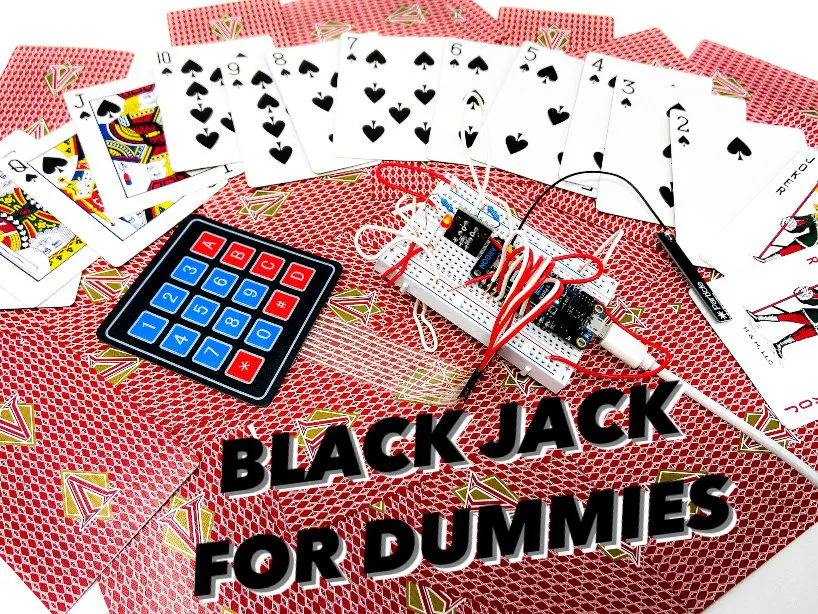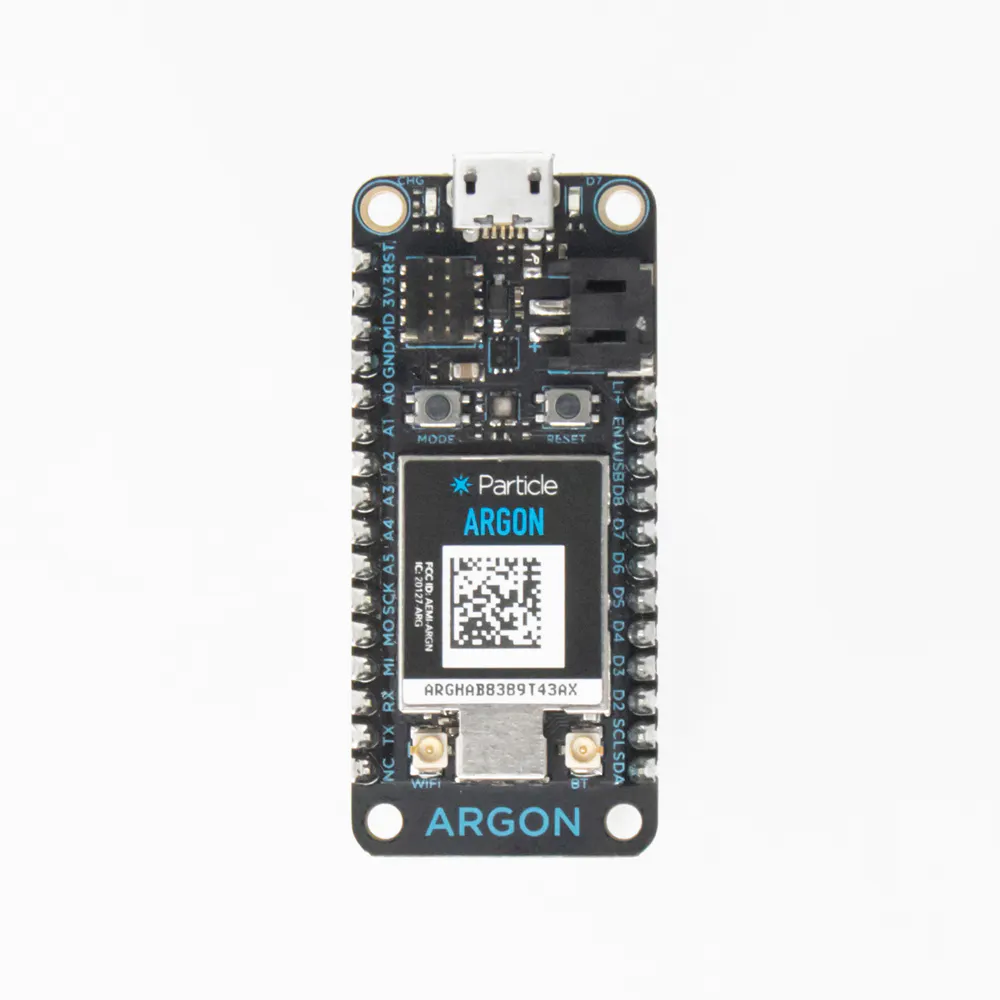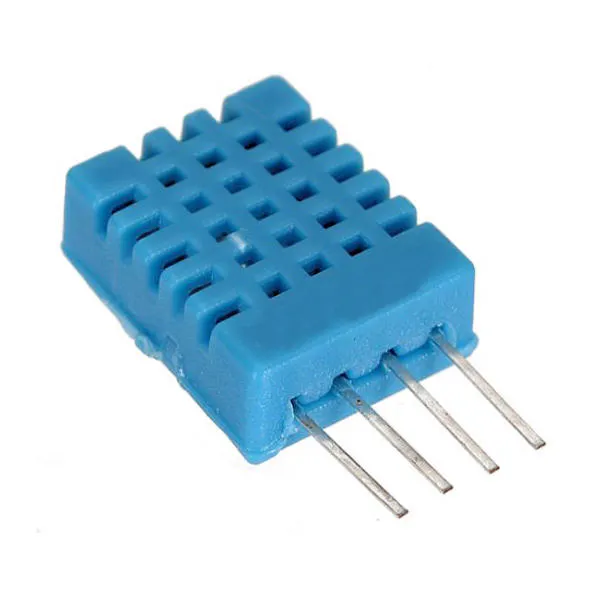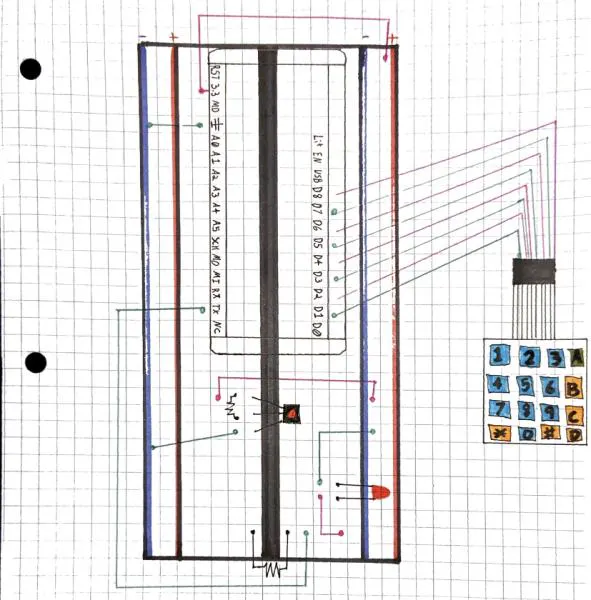# Blackjack For Dummies

A simplified game of blackjack. For all the fun and a fraction of the thought

BeginnerFull instructions provided5 hours107## Things used in this project

### Hardware componentsParticle Argon
×1DHT11 Temperature & Humidity Sensor (4 pins)
×1
 Membrane Keyboard
×1
 Button
×1

### Software apps and online servicesParticle Build Web IDEThingSpeak API

## Schematics

### Daniel Diagram## Code

### Dealer Station Code

C/C++
This code plays the roll of the dealer. It iterates through the players in the game. The players send a hit or stay option over the cloud (using Webhooks), which the dealer station receives. The dealer station then deals the player a new card if they selected hit, or passes over them if they selected stay.
```#include <iostream>
using namespace std;

void setup() {

Serial.begin(9600);

}

void loop() {
int sum = 0;
int TristanCards[] = {0,0,0,0,0};
int DanielCards[] = {0,0,0,0,0};
TristanCards = rand()%(11-1 + 1) + 1;
TristanCards = rand()%(11-1 + 1) + 1;
AdamCards = rand()%(11-1 + 1) + 1;
AdamCards = rand()%(11-1 + 1) + 1;
DanielCards = rand()%(11-1 + 1) + 1;
DanielCards = rand()%(11-1 + 1) + 1;

for (int i = 1; i <= 6; i++){
if (i==1){
int randNum = rand()%(11-1 + 1) + 1;
TristanCards = randNum;
sum = 0;
for (i = 0; i < 3; i++){
sum = TristanCards[i] + sum;
if (i==2){
if (sum > 21){
Particle.publish("Dealer Busted");
Particle.publish("sum", String(sum), PRIVATE);
}
if (sum == 21){
Particle.publish("Blackjack!", PRIVATE);
}
Particle.publish("sum", String(sum), PRIVATE);
}
}
}
if (i==2){
int press = Particle.subscribe("hook-response/buttonPressAdam", myHandler, MY_DEVICES);
if (press == 1){
int randNum = rand()%(11-1 + 1) + 1;
sum = 0;
for (i = 0; i < 3; i++){
if (i==2){
if (sum > 21){
Particle.publish("sum", String(sum), PRIVATE);
}
if (sum == 21){
Particle.publish("Blackjack!");
}
Particle.publish("sum", String(sum), PRIVATE);
}
}
}

if (i==3){
int press2 = Particle.subscribe("hook-response/buttonPressDaniel", myHandler, MY_DEVICES);
if (press2 == 1){
int randNum = rand()%(11-1 + 1) + 1;
DanielCards = randNum;
sum = 0;
for (i = 0; i < 3; i++){
sum = DanielCards[i] + sum;
if (i==2){
if (sum > 21){
Particle.publish("Daniel Busted");
Particle.publish("sum", String(sum), PRIVATE);
}
if (sum == 21){
Particle.publish("Blackjack!");
}
Particle.publish("sum", String(sum), PRIVATE);
}
}
}
}
if (i==4){
int randNum = rand()%(11-1 + 1) + 1;
TristanCards = randNum;
sum = 0;
for (i = 0; i < 4; i++){
sum = TristanCards[i] + sum;
if (i==2){
if (sum > 21){
Particle.publish("Dealer Busted");
Particle.publish("sum", String(sum), PRIVATE);
}
if (sum == 21){
Particle.publish("Blackjack!");
}
Particle.publish("sum", String(sum), PRIVATE);
}
}

}
if (i==5){
int press3 = Particle.subscribe("hook-response/buttonPressAdam", myHandler, MY_DEVICES);
if (press3 == 1){
int randNum = rand()%(11-1 + 1) + 1;
sum = 0;
for (i = 0; i < 4; i++){
if (i==2){
if (sum > 21){
Particle.publish("sum", String(sum), PRIVATE);
}
if (sum == 21){
Particle.publish("Blackjack!");
}
Particle.publish("sum", String(sum), PRIVATE);
}
}
}

}
if (i==6){
int press4 = Particle.subscribe("hook-response/buttonPressDaniel", myHandler, MY_DEVICES);
if (press4 == 1){
int randNum = rand()%(11-1 + 1) + 1;
DanielCards = randNum;
sum = 0;
for (i = 0; i < 4; i++){
sum = DanielCards[i] + sum;
if (i==2){
if (sum > 21){
Particle.publish("Daniel Busted");
Particle.publish("sum", String(sum), PRIVATE);
}
if (sum == 21){
Particle.publish("Blackjack!");
}
Particle.publish("sum", String(sum), PRIVATE);
}
}
}

}
}
}
void myHandler(const char *event, const char *data) {
// Handle the integration response
}

```

### Webhook Test Code

C/C++
This code is used to ensure that the communication between the argons is valid. It sends a value to the webhook, which can then be logged into the verify that the webhook received the value.
```void setup() {

}

void loop() {
Particle.subscribe("hook-response/buttonPressDaniel", myHandler, MY_DEVICES);
Particle.publish("sum", String(1), PRIVATE);

}
void myHandler(const char *event, const char *data) {
// Handle the integration response
}
```

### Daniel (player code)

C/C++
```// This #include statement was automatically added by the Particle IDE.

const byte ROWS = 4; //four rows
const byte COLS = 4; //four columns
//define the cymbols on the buttons of the keypads
char hexaKeys[ROWS][COLS] = {
{'1','2','3','A'},
{'4','5','6','B'},
{'7','8','9','C'},
{'*','0','#','D'}
};
byte rowPins[ROWS] = {9, 8, 7, 6}; //connect to the row pinouts of the keypad
byte colPins[COLS] = {5, 4, 3, 2}; //connect to the column pinouts of the keypad

//initialize an instance of class NewKeypad

void setup(){
Serial.begin(9600);
Particle.variable("buttonPressDaniel", hexaKeys[ROWS][COLS]);
}

void loop(){

if (customKey){
Serial.println(customKey);
Particle.publish("buttonPressDaniel", String(customKey), PRIVATE);

}
}

#define DHTPIN 9
#define DHTTYPE DHT12

DHT dht(DHTPIN, DHTTYPE);

const int TMP12Pin = A1;
const int MQ2 = A5;

double voltage, TMP12F, TMP12C, h, t, f, MQ2value;

void setup() {

Serial.begin(9600);
Serial.println("IOT PROJECT IAMS Serial Test OKAY");

pinMode(TMP12Pin, INPUT);
pinMode(MQ2, INPUT);

dht.begin();

Particle.variable("DHT-12 in degF", f);
Particle.variable("DHT-12 in degC", t);
Particle.variable("DHT-12 in %h", h);

Particle.variable("TMP12 in degF", TMP36F);
Particle.variable("TMP12 in degC", TMP36C);
Particle.variable("TMP12 volts", voltage);

}
```

C/C++
This code allows the players to utilize the membrane switch keypad to interact with the dealer. This device allows the dealer to know what action this player would like to take on his next turn.
```#include <Keypad_Particle.h>

const int ROW_NUM = 4;
const int COLUMN_NUM = 4;

char keys[ROW_NUM][COLUMN_NUM] = {
{'1','2','3','A'},
{'4','5','6','B'},
{'7','8','9','C'},
{'*','0','#','D'}
};

byte pin_rows[ROW_NUM] = {8, 7, 6, 5};
byte pin_column[COLUMN_NUM] = {4, 3, 2,1};

void setup() {

Serial.begin(9600);

}

void loop(){

if (key){
Serial.println(key);

Particle.subscribe("hook-response/sum", myHandler, MY_DEVICES);
}
}
void myHandler(const char *event, const char *data) {

}
```

## Credits

### Tristan Tyson

1 project • 3 followers